# KSEEB Solutions for Class 8 Maths Chapter 3 Axioms, Postulates and Theorems Ex 3.2

In this chapter, we provide KSEEB SSLC Class 8 Maths Chapter 3 Axioms, Postulates and Theorems Ex 3.2 for English medium students, Which will very helpful for every student in their exams. Students can download the latest KSEEB SSLC Class 8 Maths Chapter 3 Axioms, Postulates and Theorems Ex 3.2 pdf, free KSEEB SSLC Class 8 Maths Chapter 3 Axioms, Postulates and Theorems Ex 3.2 pdf download. Now you will get step by step solution to each question.

## Karnataka Board Class 8 Maths Chapter 3 Axioms, Postulates and Theorems Ex 3.2

I. Draw diagrams illustrating each of the following situations

Question a.
Three straight lines which do not pass through a fixed point.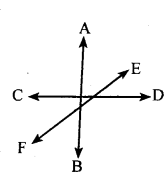Question b.
A point and rays emanating from that point such that the angle between any two adjacent rays is an acute angle.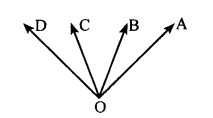Question c.
Two angles which are not adjacent angles, but still supplementary.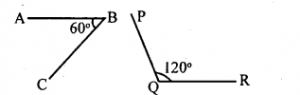Question d.
Three points in the plane which are equidistant from each other.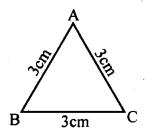Question 2.
Recognise the type of angles in the following figures.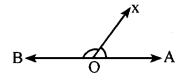AOX is an acute angle and BOX is an obtuse angle
ii. Exterior angles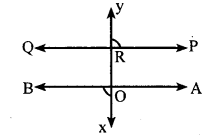PRY and BOX are supplementary angles
iii. Reflex angle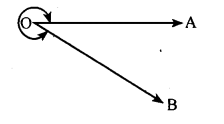Question 3.
Find the value of ‘x’ in each of the following diagrams.
(i) x + 2x = 180°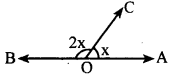3x = 180°
x = 180∘3 = 60°
∴ x= 60°
(ii) ∠COB = 90°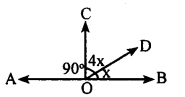∠COD +∠DOB = 90°
4x + x=90°
5x = 90
x = 905
x = 18°
∠AOC + ∠COB + ∠BOD + ∠DOA = 360° Complete angle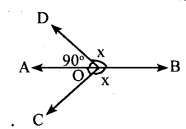∠ AOC + x + x +∠ DOA = 360°
90 + 2x = 360° [∠ AOC +∠DOA = 90° ]
2x = 360 – 90
2x = 270
x = 2702
x = 135°
(iv) ∠AOB = 180°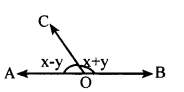∠AOC + ∠BOC = 180°
x – y + x + y = 180°
2x = 180°
x = 1802
x = 90°
(v) ∠COF= ∠EOD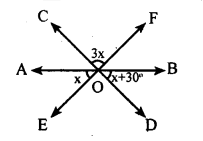∠COF = 3x
∴∠EOD = 3x
∠AOB = 180°
∠AOE + ∠EOD + ∠DOB = 180°
x + 3x + x + 30 = 180°
5x + 30 = 180°
5x = 180 – 30
5x = 150
x = 1505
x = 30°
(vi)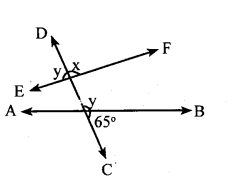EF is a straight line
∴ x + y = 180° ………..(i)
DC is a straight line
∴ y+ 65° = 180° …(ii)
From(i)and(ii)
x + y = y + 65°
x + y – y = 65°
x = 65°

Question 4.
which pair of angles are supplementary in the following diagram ? Are they supplementary rays ?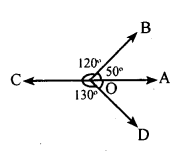∠AOB and ∠COD are supplementary angles.
∠COB and ∠AOD are supplementary angles.
There are no supplementary rays in the diagram.

Question 5.
Suppose two adjacent angles are supplementary. Show that if one of them is an obtuse angle, then the other angle must be acute.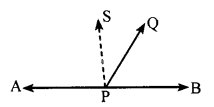In the figure
∠APQ + ∠BPQ = 180° and ∠APQ is an obtuse angle.
To prove ∠BPQ < 90°
Construction: Draw a perpendicular SP at P
Proof: In the fig
∠ APS +∠SPQ + ∠QPS = 180°
90 + ∠SPQ + ∠QPB = 180°
∠SPQ + ∠QPB = 180-90
∠SPQ +∠QPB = 90
∴∠SPQ < 90° and ∠QPB < 90°
∴∠QPB is an acute angle
∴∠APQ is an obtuse angle

All Chapter KSEEB Solutions For Class 8 maths

—————————————————————————–

All Subject KSEEB Solutions For Class 9

*************************************************

I think you got complete solutions for this chapter. If You have any queries regarding this chapter, please comment on the below section our subject teacher will answer you. We tried our best to give complete solutions so you got good marks in your exam.

If these solutions have helped you, you can also share kseebsolutionsfor.com to your friends.

Best of Luck!!